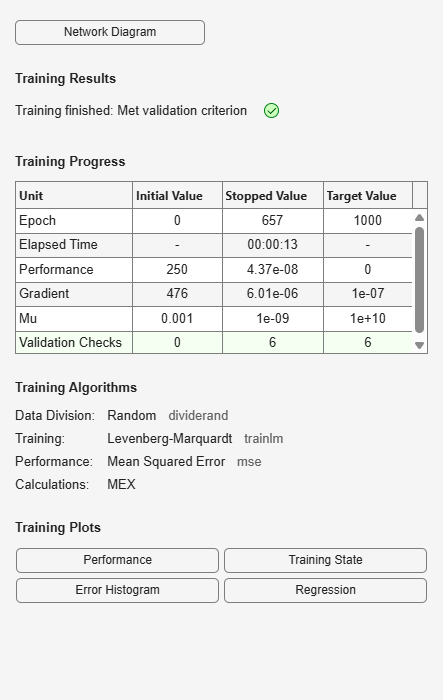# regression

(Not recommended) Perform linear regression of shallow network outputs on targets

`regression` is not recommended. Use `fitlm` (Statistics and Machine Learning Toolbox) instead. For more information, see Compatibility Considerations.

## Syntax

``[r,m,b] = regression(t,y)``
``[r,m,b] = regression(t,y,'one')``

## Description

example

````[r,m,b] = regression(t,y)` calculates the linear regression between each element of the network response and the corresponding target. This function takes cell array or matrix target t and output y, each with total matrix rows of N, and returns the regression values, r, the slopes of regression fit, m, and the y-intercepts, b, for each of the N matrix rows.```
````[r,m,b] = regression(t,y,'one')` combines all matrix rows before regressing, and returns single scalar regression, slope, and offset values.```

## Examples

collapse all

This example shows how to train a feedforward network and calculate and plot the regression between its targets and outputs.

`[x,t] = simplefit_dataset;`

The 1-by-94 matrix `x` contains the input values and the 1-by-94 matrix `t` contains the associated target output values.

Construct a feedforward neural network with one hidden layer of size 20.

`net = feedforwardnet(20);`

Train the network `net` using the training data.

`net = train(net,x,t);`

Estimate the targets using the trained network.

`y = net(x);`

Calculate and plot the regression between its targets and outputs.

`[r,m,b] = regression(t,y)`
```r = 1.0000 ```
```m = 1.0000 ```
```b = 1.0878e-04 ```
`plotregression(t,y)`## Input Arguments

collapse all

Network targets, specified as a matrix or cell array.

Network outputs, specified as a matrix or cell array.

## Output Arguments

collapse all

Regression value, returned as a scalar.

Slope of regression fit, returned as a scalar.

Offset of regression fit, returned as a scalar.

## Compatibility Considerations

expand all

Not recommended starting in R2020b